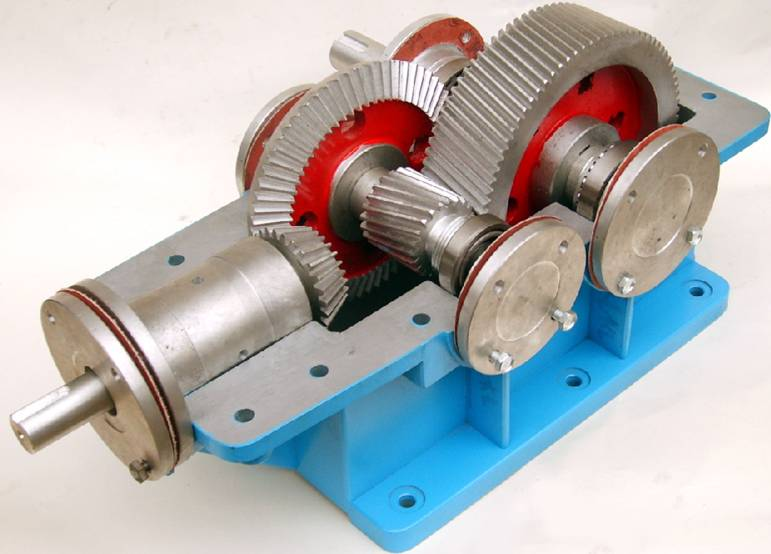021-56327980
Email：bocai178@126.com

拆装实验用大型减速器

拆装减速器制作材料除标准件外，全部用优质铝合金精加工制作而成，传动齿轮用滚齿机加工。精度高、配合正确、传动灵活，减速器外壳表面进行烤漆处理。产品精久耐用，全铝减速器我厂独家生产历史已有多年。

①、 本系列大型拆装减速器专供学生作拆装实验用，为设计和测量零件尺寸和绘制正确零部件及装配图提供了实物依据。
②、 该系列产品品种齐全、尺寸合适、重量轻、设计结构合理牢固。
③、 减速器附件类型多，开扩了学生的视野。
 编号 名 称 单位 数量 参数及尺寸 1 拆装用双级同轴式圆柱齿轮减速器 台 1 m=3 Z1=22 Z3=78 Z2=26 Z4=82 ɑ=20° β=15°a=160.5  i=11.1818 h=159mm    外形尺寸:560㎜×420㎜×350㎜ 重量:24.8KG （全部铝制） 2 拆装用双级展开式圆柱齿轮减速器 台 1 Z1=16 Z2=47 m=3  Z3=27 Z4=68 а1=99 ɑ=20°β15°а2=147 i=7.398 h=146mm   外形尺寸:535㎜×330㎜×294㎜ 重量:21KG （全铝制） 3 拆装用双级圆锥圆柱齿轮减速器 台 1 m=3 Z1=20 Z2=50 Z3=16 Z4=68 i=10.625 h=141mm a=130.5 β=15° 外形尺寸:550㎜×460㎜×360㎜ 重量:20KG （全部铝制） 4 拆装用单级圆锥齿轮减速器 台 1 m=3 Z1=20 Z2=60 ɑ=20°β15°i=3 h=129mm 外形尺寸:500×350×315     重量:15.5KG （全铝制） 5 拆装用单级圆柱齿轮减速器 台 1 m=3 Z1=72 Z2=29 ɑ=20°β=15°a=158  i=2.49  h=151mm 外形尺寸:450㎜×400㎜×340㎜ 重量:17.5KG （全部铝制） 6 拆装用蜗轮蜗杆减速器 台 1 z1=1 q=12 z2=45 m=4㎜   i=45   H=163 外形尺寸：420㎜×320㎜×400㎜ 重量 15.5KG （全部铝制） 7 拆装用新型结构单级圆柱齿轮减速器 台 1 m=2 Z1=25 Z2=75  i=3   h=120 外形尺寸：420㎜×320㎜×400㎜   （全铝制） 8 拆装用双级分流式圆柱齿轮减速器 台 1 m=3 Z1=16 Z2=47 Z3=27 Z4=68 i=7.398 а1=99 ɑ=20° h=146mmа2=147 β15°  外形尺寸:540㎜×360㎜×320㎜ 重量:24.5KG    （全部铝制）

BCJSX-C型系列 拆装实验用大型减速器QQ：438831120 1601241314

Email：bocai178@126.com